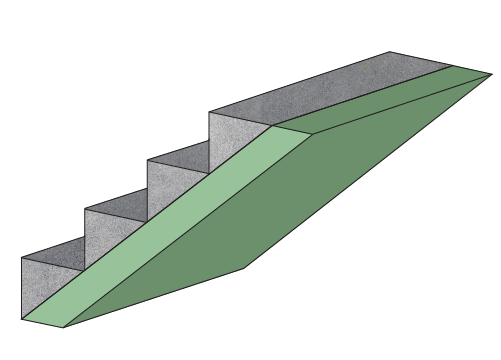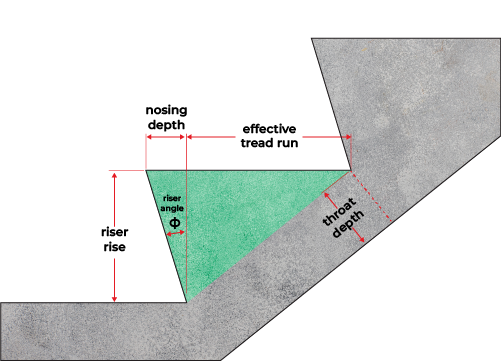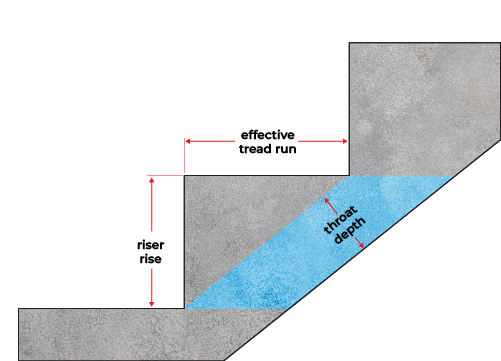# Concrete Stairs Calculator

Constructing concrete stairs will be easier with this concrete stairs calculator! Find out how much concrete you'll need when building one before or... Read more

2. How to calculate stairs' concrete volume
3. How to use this concrete stairs calculator
4. FAQs

Before we learn how to calculate the stairs' concrete volume, we first need to know the different parts of a stair. Though we have a separate stair calculator that tackles that, here we will introduce some other stair dimensions: the stairs' carriage and throat depth.

Though the carriage may resemble a stair stringer, they are now different since we're dealing with concrete stairs.

Apart from the common step's riser and run (which are the distance between two adjacent steps, vertically and horizontally, respectively), we'll also need to consider the carriage because that connects the steps together, as shown colored light green in the illustration below:The carriage will also encase the reinforcing steel bars when needed, especially if you want to keep a storage space underneath the stairs.

On the other hand, the throat depth is basically the thickness of the carriage. It is also the perpendicular distance between the underside of the carriage and the inner joint between a tread and its consecutive riser.

Now that we've discussed the different details of the stairs we need for the basic calculation of the stairs' concrete, let's now learn how to calculate how much volume of concrete we'll need for a concrete stair project.

Check other Cement and concrete calculators 🏦

## 2. How to calculate stairs' concrete volume

Considering a flight of stairs as a prism makes calculating its volume easy. We can break down our flight of stairs into parts to get its cross-sectional or end area and then multiply it by its width to find its volume.

Let's start by finding the end area of a step for a stair with a regular vertical riser, highlighted in yellow in the image below:We obtain that area using the formula shown below:

$\footnotesize \begin{gather*}\rm basic\ step\\[-5px]\rm area \end{gather*} = \frac{\begin{gather*}\rm effective\\[-5px]\rm tread\ run \end{gather*} \times \begin{gather*}\rm riser\\[-5px]\rm rise \end{gather*}}{2}$On the other hand, for the stairs with slanted or angled risers shown in the image above highlighted in green, we can use this general step area formula:

$\footnotesize \begin{gather*}\rm step\\[-5px]\rm area \end{gather*} = \frac{\left(\begin{gather*}\rm effective\\[-5px]\rm tread\ run \end{gather*} + \begin{gather*}\rm nosing\\[-5px]\rm depth \end{gather*}\right)\! \times\! \begin{gather*}\rm riser\\[-5px]\rm rise \end{gather*}}{2}$

We can also use this formula if we prefer to indicate the riser angle, $\theta$:

$\scriptsize \begin{gather*}\rm step\\[-5px]\rm area \end{gather*}\! =\! \frac{\left(\begin{gather*}\rm effective\\[-5px]\rm tread\\[-5px]\rm run \end{gather*}\! + \begin{gather*}\rm riser\\[-5px]\rm rise \end{gather*}\! \times \tan(\theta)\right)\!\! \times\! \begin{gather*}\rm riser\\[-5px]\rm rise \end{gather*}}{2}$

After obtaining the step area, we then determine the parallelogram area of the carriage's portion under each step, as shown highlighted in blue in the image below:To get that area, we begin by using the Pythagorean theorem to obtain the diagonal that connects the inner joints of each step and then multiplying that length by the throat depth of the carriage, as we can see in the equation below:

$\scriptsize \begin{gather*}\rm carriage\\[-5px]\rm portion\\[-5px]\rm area \end{gather*} = \sqrt{\begin{gather*}\rm effective\\[-5px]\rm tread\\[-5px]\rm run \end{gather*}^2 + \begin{gather*}\rm riser\\[-5px]\rm rise \end{gather*}^2} \times \begin{gather*}\rm throat\\[-5px]\rm depth \end{gather*}$

To get the total end area of a flight of stairs, we multiply the sum of the step area and the carriage portion area by the number of steps, as shown in this formula:

$\scriptsize \begin{gather*}\rm total\ end\\[-5px]\rm area \end{gather*} =\! \begin{gather*}\rm number\\[-5px]\rm of\ steps \end{gather*} \times\!\! \left(\begin{gather*}\rm step\\[-5px]\rm area \end{gather*} +\! \begin{gather*}\rm carriage\\[-5px]\rm portion\\[-5px]\rm area \end{gather*}\right)$

Lastly, we multiply this area by the stair width to get the stair volume, shown below:

$\footnotesize \begin{gather*}\rm stair\\[-5px]\rm volume \end{gather*} =\! \begin{gather*}\rm total\ end\\[-5px]\rm area \end{gather*} \times \begin{gather*}\rm stair\\[-5px]\rm width \end{gather*}$

That's it! We can now use that volume for the concrete volume we need to pour into our concrete stairs formwork.

## 3. How to use this concrete stairs calculator

Though the formulas presented above are easy to follow, performing multiple stairs concrete calculations can also be tiring. That's why we made this concrete stairs calculator for you. Here are the steps on how to use it:

1. Enter the number of steps of your flight of stairs.

2. Key in the effective tread run and the riser rise of your steps.

3. If you want or need to calculate the concrete volume for stairs with angled risers, select Yes for the "Do you want angled risers" question. That will display the nosing depth and the riser angle. You need to input one of them, whichever is known to you.

4. Finally, enter the width of your stairs.

After these steps, you'll see the concrete volume you'll need for your concrete stairs.

We've also included a wastage feature so you can enter how much concrete percentage of your needed concrete you anticipate wasting during concrete pouring. We labeled the outcome volume, including the wastage, as the concrete volume to purchase.

✅ Looking ahead, you might also want to check our stair carpet calculator if you plan to put one on your stairs.

4. FAQs

### What is the formula to calculate concrete stairs volume?

The formula to calculate concrete stairs volume is total end area × stair width. We calculate the total end area by using this equation:

A = n × (r × t / 2 + √(r² × t²) × d)

where:

• A — Total end area;
• n — Number of steps;
• r — Riser rise;
• t — Effective tread run; and
• d — Throat depth.

### How do I find the volume of a concrete stair?

To find the volume of a concrete stair, let's say with four steps, a 20-cm riser rise, a 25-cm effective tread run, a 10-cm throat depth, and a 100-cm stair width, we do the following:

1. Multiply the riser rise and the effective tread run and divide the answer by two, as shown here:

20 cm × 25 cm / 2 = 250 cm²

2. Take the square root of the sum of the squares of the effective tread run and the riser rise to get:

√((20 cm)² + (25 cm)²) = √(400 cm² + 625 cm²) = √1025 cm²
= 32.02 cm

3. Multiply that length by the throat depth to find the area of that portion under a step:

32.02 cm × 10 cm = 320.2 cm²

4. Add the end area of the step to that area and multiply the sum by the number of steps to get the total end area, as shown here:

(320.02 cm² + 250 cm²) × 4
= 2,280.08 cm²

5. Finally, multiply the total end area by the stair width for your needed concrete volume:
2,280.08 cm² × 100 cm
= 228,008 cm³

### Do I need to reinforce my concrete stairs with rebars?

Yes, if you're building a floating staircase where you'll want a space underneath it, and no, if you'll make a staircase over a backfill composed of compacted soil.

Concrete is durable under compression, meaning it can surpass extreme compressive loads. However, concrete needs the help of reinforcing steel bars to sustain flexural or bending forces.

### What is the common width of stairs?

We commonly construct stairs 3 feet (or approximately 0.9 meters) wide or more. We follow that standard width of stairs to safely accommodate two persons crossing each other on a flight of stairs.